# Radiative Heat Transfer (Part - 4) Chemical Engineering Notes | EduRev

## Chemical Engineering : Radiative Heat Transfer (Part - 4) Chemical Engineering Notes | EduRev

The document Radiative Heat Transfer (Part - 4) Chemical Engineering Notes | EduRev is a part of the Chemical Engineering Course Heat Transfer for Engg..
All you need of Chemical Engineering at this link: Chemical Engineering

7.4 Radiative heat exchanger between surfaces
Till now we have discussed fundamental aspects of various definitions and laws. Now we will study the heat exchange between two or more surfaces which is of practical importance. The two surfaces which are not in direct contact, exchanges the heat due to radiation phenomena. The factors those determine the rate of heat exchange between two bodies are the temperature of the individual surfaces, their emissivities, as well as how well one surface can see the other surface. The last factor is known as view factor, shape factor, angle factor or configuration factor.

7.4.1 View factor

In this section we would like to find the energy exchange between two black surfaces having area A1 and A2, respectively, and they are at different temperature and have arbitrary shape and orientation with respect to each other. In order to find the radiative heat exchange between the bodies we have to first define the view factor as

F12 = fraction of the energy leavings surface 1 which reaches surface 2

F21 = fraction of the energy leaving surface 2 which reaches surface 1 or in general,

Fmn = fraction of the energy leaving surface m which raches surface n

Thus the energy leaving surface 1 and arriving at surface 2 is Eb1A1F12 and the energy leaving surface 2 and arriving at surface 1 is Eb2A2F21. All the incident radiation will be absorbed by the blackbody and the net energy exchange will be,

Q = Eb1A1F12 - Eb2A2F21

At thermal equilibrium between the surfaces Q12 = 0 and Eb1 = Eb2, thus

0 = Eb1 (A1F12 - A2F21)

A1F12 - A2F21                     (7.19

Equation 7.19 is known as reciprocating relation, and it can be applied in general way for any blackbody surfaces.

AiFij - AjFji                    (7.20)

Though the relation is valid for blackbody it may be applied to any surface as long as diffuse radiation is involved.

7.4.1.1 Relation between view factors

In this section we will develop some useful relation of view factor considering fig. 7.5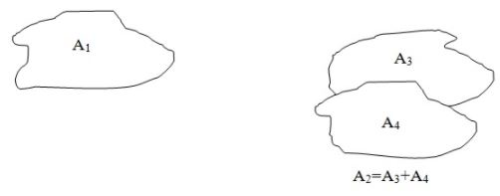Fig. 7.5: Exchange of energy between area A1 and A2 (A is area of blackbody)

View factor for radiation from A1 to the combined area A2,

F12 = F13 + F14              (7.21)

and using the reciprocating relations for surface 1 and 4,

A1F14 = A4F41                     (7.22)

Using eq. 7.21 and 7.22,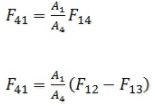Thus the unknown view factor F14 can be estimated if the view factors F12 and F13, as well as their areas are (A1, A2) known.

Now, consider a flat plate (for eg.) which is emitting the radiation, it can be understood that the radiation of the flat plat cannot fall on its own surface (partly or fully). Such kind or surfaces are termed as “not able to see itself”. In such situations,

F11 = F22 = F33 = F44 = 0

However, if the surface can see itself like concave curved surfaces, which may thus see themselves and then the shape factor will not be zero in those cases.

Another property of the shape factor is that when the surface is enclosed, then the following relation holds,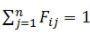(7.23)

where, Fij is the fraction of the total energy leaving surface i which arrives at surface j.

In case of N-walled enclosure, some of the view factors may be evaluated from the knowledge of the rest and the total N2 view factors may be represented in square matrix form shown below,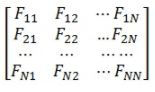Illustration 7.2

A pipe having 10 cm of diameter is carrying saturated steam at 8 bar of absolute pressure. The pipe runs through a room. The wall of the room is at 300 oK. A portion around 1 m of the pipe insulation is damaged and exposed to the room atmosphere. Calculate the net rate of heat loss from the pipe by radiation.

Solution 7.2

The emissivity of the pipe surface is not given so it may be considered black.  Moreover, since the room may be big compared to the surface area of the pipe, the room may also be considered to be a blackbody.
We can write F11 + F12 = 1.
The value of F11 = 0, as the pipe cannot see itself.
Thus F12, the view factor (1-pipe, 2-room) will be 1.
The net rate heat loss due to radiation,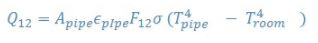Tpipe can be obtained by the temperature of the steam at the prevailing pressure with the help of steam table = 450 K.

σ (= 5.67 X 10-8 W/m2/K4)

On putting the value,

Q12 = {π(0.1)(1)}(1)(1)(5.67 X 10-8){4504 - 3004}

Q12 = 586 W

7.5 Heat exchange between non blackbodies
Evaluation of radiative heat transfer between black surfaces is relatively easy because in case of blackbody all the radiant energy which strikes the surface is absorbed. However, finding view factor is slightly complex, but once it can be done, finding heat exchange between the black bodies is quite easy.

When non blackbodies are involved the heat transfer process becomes very complex because all the energy striking on to the surface does not get absorbed. A part of this striking energy reflected back to another heat transfer surface, and part may be reflected out from the system entirely. Now, one can imagine that this radiant energy can be reflected back and forth between the heat transfer surfaces many times.

In this section, we will assume that all surfaces are in the analysis are diffuse and uniform in temperature and that the reflective and emissive properties are constant over all surfaces.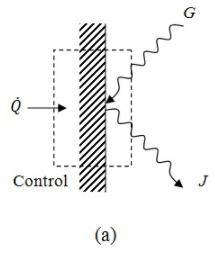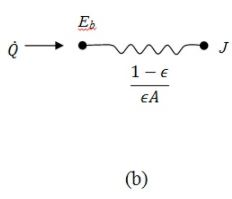Fig. 7.6: (a) Surface energy balance for opaque surface (b) equivalent electrical circuit

It is also assumed that the radiosity and irradiation are uniform over each surface. As we have already discussed that the radiosity is the sum of the energy emitted and the energy reflected when no energy is transmitted (as opaque body), or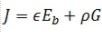(7.24)

where, ∈ is the emissivity and Eb is the blackbody emissive power. Because the transmissivity is zero due to opaque surface and absorptivity of the body (grey) will be equal to its emissivity by Kirchhoff’s law.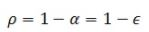Thus, eq.7.24 becomes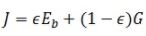(7.25)

The net energy leaving the surface is the difference between the radiosity and the irradiance (fig.7.6a),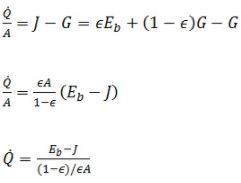(7.26)

The eq.7.26 can be analogous to the electrical circuit as shown in fig.7.6(b). The numerator of the eq.7.26 is equivalent to the potential difference, denominator is equivalent to the surface resistance to radiative heat, and left part is equivalent to the current in the circuit.

In the above discussion we have considered only one surface. Now we will analyse the exchange of radiant energy by two surfaces, A1 and A2, as shown in the fig.7.7a.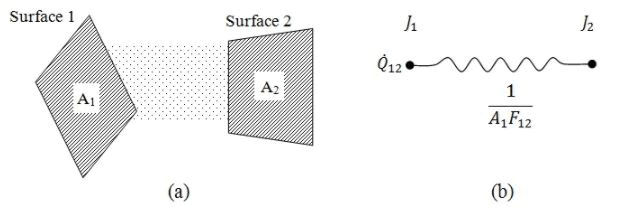Fig. 7.7: (a) Energy exchange between two surfaces, (b) equivalent circuit diagram

The radiation which leaves surface 1, the amount that reaches surface 2 is

J1A1F12

Similarly, the radiation which leaves system 2, the amount that reaches surface 1 is

J2A2F21

The net energy transfer between the surfaces,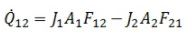Reciprocity theorem states that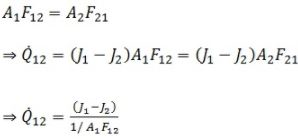(7.27)

It also resembles an electrical circuit shown in fig.7.7b. The difference between eq.7.26 and 7.27 is that in eq.7.27 the denominator term is space resistance instead of surface resistance.

Now, to know, the net energy exchange between the two surfaces we need to add both the surface resistances along with the overall potential as shown in the fig.7.8. Here the surfaces see each other and nothing else.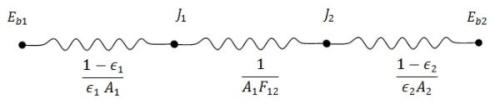Fig. 7.8: Radiative nature for two surfaces which can see each other nothing else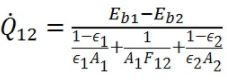(7.28)

Offer running on EduRev: Apply code STAYHOME200 to get INR 200 off on our premium plan EduRev Infinity!

## Heat Transfer for Engg.

46 videos|60 docs|59 tests

,

,

,

,

,

,

,

,

,

,

,

,

,

,

,

,

,

,

,

,

,

;